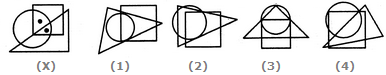# Non Verbal Reasoning - Dot Situation - Discussion

### Discussion :: Dot Situation - Section 1 (Q.No.1)

From amongst the figures marked (1), (2), (3) and (4), select the figure which satisfies the same conditions of placement of the dots as in figure (X).

1.

Select the figure which satisfies the same conditions of placement of the dots as in Figure-X.[A]. 1 [B]. 2 [C]. 3 [D]. 4

Explanation:

In fig. (X), one of the dots lies in the region common to the circle and the square only and the other dot lies in the region common to all the three figures -the circle, the square and the triangle. In each of the alternatives (1), (2) and (3), there is no region common to the square and the circle only. Only fig. (4) consists of both the types of regions.

 Budhaditya said: (Jul 23, 2016) The answer is also be option A.

 Nure Asrafi said: (Jan 6, 2017) In the figure x, the triangle is a right angle triangle so it should be 1 in answer in-spite of 4.

 Devi said: (Dec 8, 2017) Anyone, please explain me to solve this type of questions.

 Amir Sohel said: (May 16, 2018) As per given figure X, one Dot lies at the common region of circle and square. And another at the common region of all three. And these two dots are separated by one side of the triangle. That's why the answer is 4.

 Amir Sohel said: (May 16, 2018) As per the Figure X. Out of two dots, one lies at the common region of circle & square and another at the common region of circle, square and triangle. And also these dots are separated by one side(you can say larger side) of the triangle. It could be made in figure 4 only.

 Siva said: (May 16, 2020) It should be option A. I too agree.

 Lalitha said: (Aug 2, 2021) Yes, it is option A.